# A.1.4.2 Matching Distributions

Which dot plot represents the same data as the histogram below?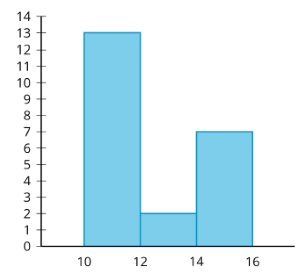Select all that apply
• A
• B
• C

Which dot plot represents the same data as the histogram below?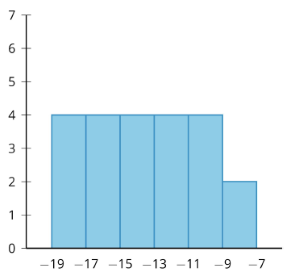Select all that apply
• A
• B
• C

Which dot plot represents the same data as the histogram below?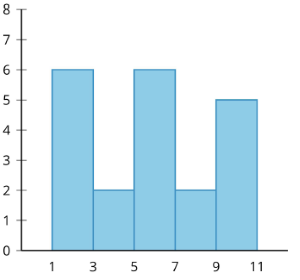Select all that apply
• A
• B
• C

Which dot plot represents the same data as the histogram below?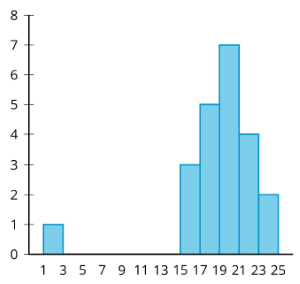Select all that apply
• A
• B
• C

Which dot plot represents the same data as the histogram below?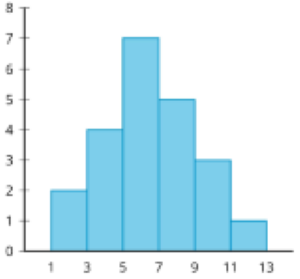Select all that apply
• A
• B
• C